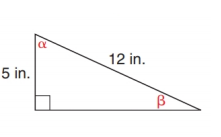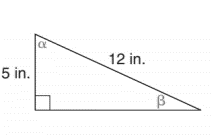Chapter 11.2, Problem 27E### Elementary Geometry for College St...

6th Edition
Daniel C. Alexander + 1 other
ISBN: 9781285195698

#### Solutions

Chapter
Section### Elementary Geometry for College St...

6th Edition
Daniel C. Alexander + 1 other
ISBN: 9781285195698
Textbook Problem
1 views

# In Exercise 23 to 28, use either the sine ratio or the cosine ratio as needed to find the measure of each indicated angle to the nearest degree.To determine

To find:

The measure of each indicated angle of the following triangle,Explanation

Consider the following figure,

General formula for cosine ratio and sin ratio is given below,

From the given figure the value 12 in. is the hypotenuse value since it is opposite to right angle.

Then 5 in. is the length of the leg adjacent to α and 5 in. is the length of leg opposite to β.

Therefore,

### Still sussing out bartleby?

Check out a sample textbook solution.

See a sample solution

#### The Solution to Your Study Problems

Bartleby provides explanations to thousands of textbook problems written by our experts, many with advanced degrees!

Get Started

#### Evaluate the indefinite integral. 5tsin(5t)dt

Single Variable Calculus: Early Transcendentals, Volume I

#### Convert the expressions in Exercises 8596 radical form. x4/3

Finite Mathematics and Applied Calculus (MindTap Course List)

#### True or False: is a geometric series.

Study Guide for Stewart's Single Variable Calculus: Early Transcendentals, 8th

#### What is the goal of a hypothesis test?

Research Methods for the Behavioral Sciences (MindTap Course List)